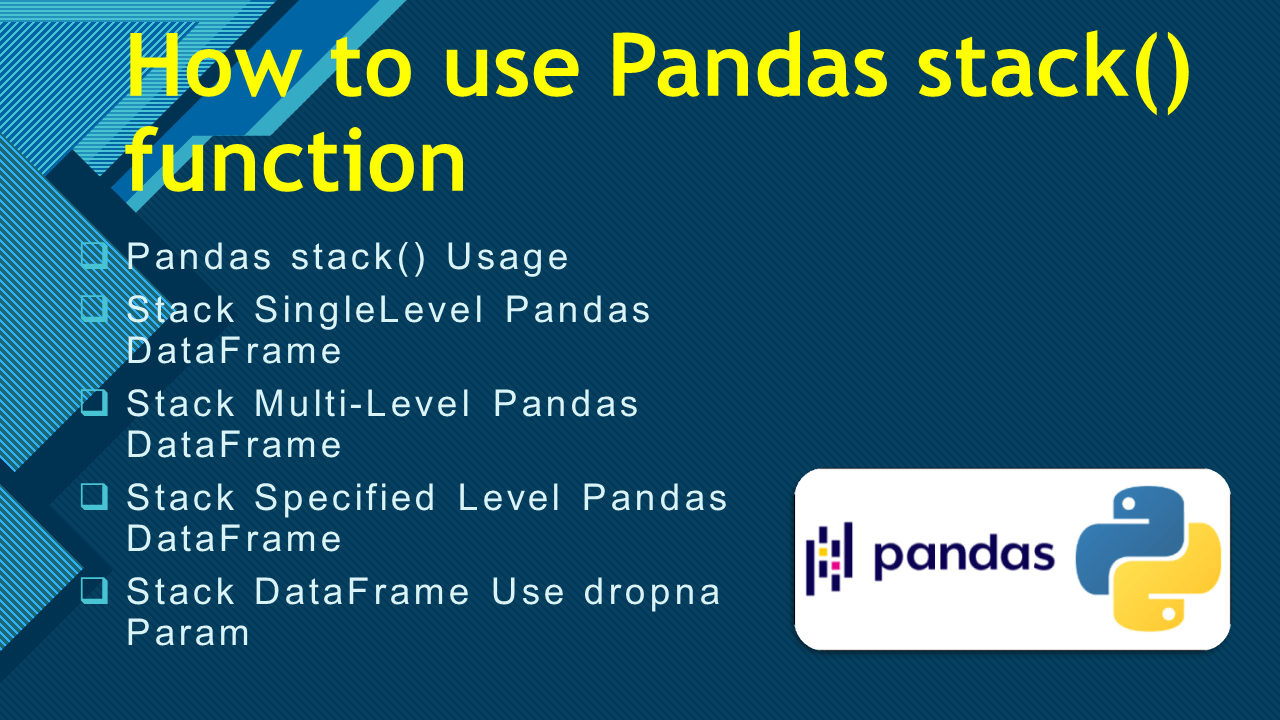# How to use Pandas stack() function

• Post author:
• Post category:Pandas

`Pandas.DataFrame.stack()` function is used to reshape the given DataFrame by transposing specified column level into row level. By default, it transposes the innermost column level. This is one of the techniques for reshaping the DataFrame. When we want to analyze or reshape, Pandas provides in-built functions. Among those functions `stack()` and `unstack()` functions are the most popular functions for transposing row level to column level and vice versa.

In this article, I will explain the Pandas `stack()` function and using this syntax and parameters how we can transpose the single or multi-level column to row level with examples.

## 1. Quick Examples of stack() function

If you are in a hurry, below are some quick examples of Pandas stack() function.

``````
# Below are the quick examples

# Example 1: Apply stack on single level column DataFrame
df = pd.DataFrame([["30-12-2010", 40.7],
["31-12-2010", 40.5]],
columns = ["Date","Temp"],
index = ['Seattle', 'Sanfrancesco']
)
print(df)
print("Stacked DataFrame:\n", df.stack())

# Example 2: Stack the multi-level DataFrame
multi_col = pd.MultiIndex.from_tuples(
[('Temp', 'Min'), ('Temp', 'Max')]
)
df = pd.DataFrame(
[[38.2, 40.1], [40.4, 43.3]],
index =  ['Seattle', 'Sanfrancesco'],
columns = multi_col
)
print(df)
print("Stacked DataFrame:\n", df.stack())

# Example 3: Stack the multi-level DataFrame
multi_col1 = pd.MultiIndex.from_tuples(
[('Temp', 'Min'), ('Wind', 'Mph')]
)
df = pd.DataFrame(
[[38.6, 8], [40.2, 6]],
index =  ['Seattle', 'Sanfrancesco'],
columns=multi_col1
)
print(df)
print("Stacked DataFrame:\n", df.stack())

# Example 4: Stack specified level DataFrame
print("Stacked DataFrame:\n", df.stack(level = 0))

# Example 5: Use Dropna param in stacking
print("Stacked DataFrame:\n", df.stack(dropna = False))
``````

## 2. Syntax of Pandas stack()

Following is the syntax of unstack() function.

``````
# Syntax of Pandas stack()
DataFrame.stack(level=- 1, dropna=True)
``````

### 2.1 Parameters

Following are the parameters of stack() function. It has two parameters.

• `level :` (int, str, list of int, and list of str)By default it is -1 i.e. last level can be stacked. If we pass specified level, it will stack those level from the column level to the row level.
• `dropna :` (bool, default True) Is used to manage the NaN values which are formed by stacking of DataFrame.

### 2.2 Return Value

It returns a stacked Series or a DataFrame.

## 3. Pandas stack() Usage

In Pandas `df.stack()` function reshapes the given DataFrame by converting the column label to a row index. It returns a Series object. It is transposed form of the original DataFrame. This function is reverse to unstack() function where stacking is done from row level to column level.

### 3.1 Stack SingleLevel Pandas DataFrame

Let’s create DataFrame with a single level column and apply the Pandas `stack()` function, it will return the stacked Pandas Series.

``````
# Create DataFrame with single level column
import pandas as pd
df = pd.DataFrame([["30-12-2010", 40.7],
["31-12-2010", 40.5]],
columns = ["Date","Temp"],
index = ['Seattle', 'Sanfrancesco']
)
print(df)
# Apply stack on single level column DataFrame
print("Stacked DataFrame:\n", df.stack())
``````

Yields below output.

# 4. Stack Multi-Level Pandas DataFrame

Using `stack()` function we can reshape the DataFrame that has multi-level columns. Pandas provide the easiest way to create multi indexes DataFrame for both column and row using pandas.MultiIndex.from_tuples().

Let’s use this function to add the multi-level columns to DataFrame and apply the stack() function to it. By default, it will stack the innermost column level into row level.

``````
# Stack the Multi-level DataFrame
multi_col = pd.MultiIndex.from_tuples(
[('Temp', 'Min'), ('Temp', 'Max')]
)
df = pd.DataFrame(
[[38.2, 40.1], [40.4, 43.3]],
index =  ['Seattle', 'Sanfrancesco'],
columns = multi_col
)
print(df)
print("Stacked DataFrame:\n", df.stack())
``````

Yields below output.

``````
# Output:
Temp
Min   Max
Seattle       38.2  40.1
Sanfrancesco  40.4  43.3

Stacked DataFrame:
Temp
Seattle      Max  40.1
Min  38.2
Sanfrancesco Max  43.3
Min  40.4
``````

## 5. Stack Specified Level Pandas DataFrame

As we know from the above, by default(level = -1) it will stack the innermost column level. When we want to stack a specified level we have to set `level` param with a specified level or list of levels. It will stack specified column level to row level. For example,

``````
# Stack specified level DataFrame
multi_col1 = pd.MultiIndex.from_tuples(
[('Temp', 'Min'), ('Wind', 'Mph')]
)
df = pd.DataFrame(
[[38.6, 8], [40.2, 6]],
index =  ['Seattle', 'Sanfrancesco'],
columns=multi_col1
)
print(df)
print("Stacked DataFrame:\n", df.stack(level = 0))
``````

Yields below output.

``````
# Output:
Temp Wind
Min  Mph
Seattle       38.6    8
Sanfrancesco  40.2    6

Stacked DataFrame:
Min  Mph
Seattle      Temp  38.6  NaN
Wind   NaN  8.0
Sanfrancesco Temp  40.2  NaN
Wind   NaN  6.0
``````

## 6. Stack DataFrame Use dropna Param

By default, while stacking we can get a row with NaN values. To overcome this problem set `False` to `dropna` Param.

``````
# Use Dropna param in stacking
multi_col1 = pd.MultiIndex.from_tuples(
[('Temp', 'Min'), ('Wind', 'Mph')]
)
df = pd.DataFrame(
[[None, 8], [40.2, 6]],
index =  ['Seattle', 'Sanfrancesco'],
columns=multi_col1
)
print(df)
print("Stacked DataFrame:\n", df.stack())
print("Stacked DataFrame:\n", df.stack(dropna = False))
``````

Yields below output.

``````
# Output:
Temp Wind
Min  Mph
Seattle        NaN    8
Sanfrancesco  40.2    6

Stacked DataFrame:
Temp  Wind
Seattle      Mph   NaN   8.0
Sanfrancesco Min  40.2   NaN
Mph   NaN   6.0

Stacked DataFrame:
Temp  Wind
Seattle      Min   NaN   NaN
Mph   NaN   8.0
Sanfrancesco Min  40.2   NaN
Mph   NaN   6.0
``````

## 7. Conclusion

In this article, I have explained the Pandas `stack()` function and using this syntax and parameters how we can transpose the single/multi-level column level to row level with examples.

Happy learning!!

## References

### Vijetha

With 5 of experience in technical writing, I have had the privilege to work with a diverse range of technologies like Python, Pandas, NumPy and R. During this time, I have consistently demonstrated my ability to grasp intricate technical details and transform them into comprehensible materials.Pandas stack() function